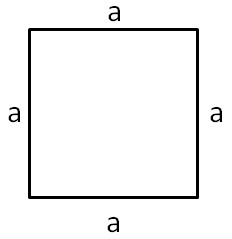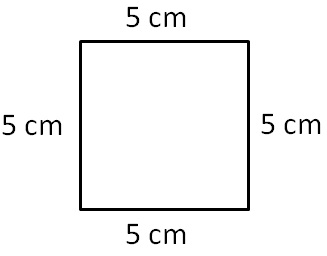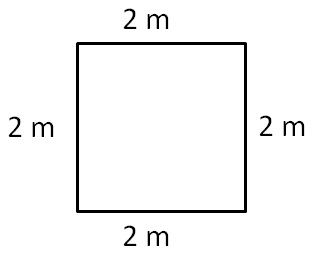We had found area of square by counting squares.

But, that method is too long

To find area of any figure,

We use formula

For a square with side aArea of square = a × a

=  a 2

Lets take a few examples

#### Find area of square with side 5 cmSide of square = a = 5 cm

Area of square = a × a

= 5 × 5

= 25 square cm

= 25 cm 2

#### Find area of square with side 2 mSide of square = a = 2 m

Area of square = a × a

= 2 × 2

= 4 square m

= 4 m 2

1. Chapter 11 Class 7 Perimeter and Area
2. Concept wise
3. Square and Rectangle

Square and Rectangle

About the AuthorDavneet Singh
Davneet Singh is a graduate from Indian Institute of Technology, Kanpur. He has been teaching from the past 9 years. He provides courses for Maths and Science at Teachoo.